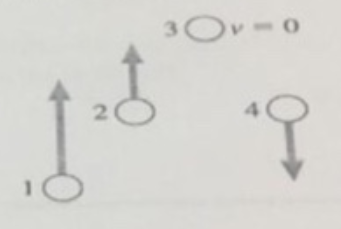# Problem: Rank in order, from largest to smallest, the gravitational potential energies of the balls.A. 1 &gt; 2 = 4 &gt; 3B. 1 &gt; 2 &gt; 3 &gt; 4C. 3 &gt; 2 &gt; 4 &gt;1D. 3 &gt; 2 = 4 &gt; 1E. None of these.

###### FREE Expert Solution

Gravitational potential energy:

$\overline{){\mathbf{P}}{{\mathbf{E}}}_{{\mathbf{g}}}{\mathbf{=}}{\mathbf{m}}{\mathbf{g}}{\mathbf{h}}}$

This implies that gravitational potential energy is directly proportional to the height.###### Problem Details

Rank in order, from largest to smallest, the gravitational potential energies of the balls.A. 1 > 2 = 4 > 3

B. 1 > 2 > 3 > 4

C. 3 > 2 > 4 >1

D. 3 > 2 = 4 > 1

E. None of these.